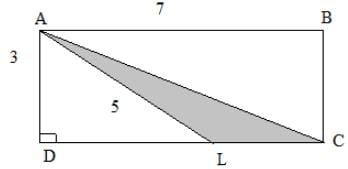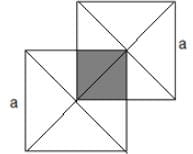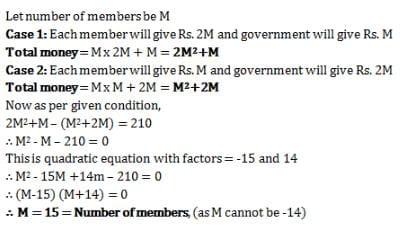Test: Problem Solving- 1

# Test: Problem Solving- 1

Test Description

## 10 Questions MCQ Test General Test Preparation for CUET | Test: Problem Solving- 1

Test: Problem Solving- 1 for SSC CGL 2023 is part of General Test Preparation for CUET preparation. The Test: Problem Solving- 1 questions and answers have been prepared according to the SSC CGL exam syllabus.The Test: Problem Solving- 1 MCQs are made for SSC CGL 2023 Exam. Find important definitions, questions, notes, meanings, examples, exercises, MCQs and online tests for Test: Problem Solving- 1 below.
Solutions of Test: Problem Solving- 1 questions in English are available as part of our General Test Preparation for CUET for SSC CGL & Test: Problem Solving- 1 solutions in Hindi for General Test Preparation for CUET course. Download more important topics, notes, lectures and mock test series for SSC CGL Exam by signing up for free. Attempt Test: Problem Solving- 1 | 10 questions in 20 minutes | Mock test for SSC CGL preparation | Free important questions MCQ to study General Test Preparation for CUET for SSC CGL Exam | Download free PDF with solutions
 1 Crore+ students have signed up on EduRev. Have you?
Test: Problem Solving- 1 - Question 1

### What is the area of triangle ALC in the figure given below?Detailed Solution for Test: Problem Solving- 1 - Question 1

First of all, we need to find LC, but for that, we need to find DL. We know that AB= DC = 7.

So, LC = DC- DL

Now, DL = √(5^2- 3^2 )= 4 (using Pythagoras Theorem)

So, LC = 7-4 = 3

Therefore, Area = (1/2)(Base)(Altitude) = (0.5)(3)(3) = 4.5

Test: Problem Solving- 1 - Question 2

### Alan has two more than twice as many chocolates as does Alice, and half as many chocolates as does Nadia. If Alice has ‘a’ number of chocolates, then in terms of ‘a’, how many chocolates do Alan, Alice and Nadia have?

Detailed Solution for Test: Problem Solving- 1 - Question 2

We know that Alice has 'a' chocolates. Alan has 2a+2 chocolates from the given statement in the question. Nadia has double the chocolates as Alan has, so she has 4a+4 chocolates.

Adding these, we get 4a+4+2a+2+a = 7a +6 . So ,Option (d) is correct.

Test: Problem Solving- 1 - Question 3

### Milk needs to be thinned to a ratio of 3 parts milk to 2 parts water. The milk-man has by mistake added water so that he has 8 liters of milk which is half water and half milk. What must he add to make the proportions of the mixture correct?

Detailed Solution for Test: Problem Solving- 1 - Question 3

We note that the final ratio must be 3:2 for milk and water respectively. Now, according to given scenario in the question, we have eight liters of solution with 4 liters milk and 4 liters water, which makes it 2:2. In order to make it 3:2, we add 2 liters milk. This would make a total of 6 liters milk and 4 liters water i.e. 6:4 which can be simplified to 3:2.

Test: Problem Solving- 1 - Question 4

The width of a rectangle is 2/3 times its length. If the length is calculated to be 9, what is the value of perimeter for this rectangle?

Detailed Solution for Test: Problem Solving- 1 - Question 4

As the width of the rectangle is 2/3 times its length which is 9, the width comes out to be 6. Therefore, the perimeter becomes, 6+6+9+9 = 30. (Perimeter of a rectangle is calculated by adding the lengths of each side of that rectangle)

Test: Problem Solving- 1 - Question 5

A line l is parallel to the y-axis and passes through the point (2,3). What is its gradient (m) and x-intercept?

Detailed Solution for Test: Problem Solving- 1 - Question 5

As the given line is parallel to the y-axis, and the slope of y-axis is infinite, therefore its slope is also infinite. As the line is defined by x=2, hence its x intercept will also be 2 because all of its points will be of the form (2,y).

Test: Problem Solving- 1 - Question 6

What is the equation of the new parabola created by shifting y = x2 , three units in the positive y-axis direction?

A. y = (x+3)2

B. y = x2

C. y = x2 + 3

D. 3y = x2

E. y = x3

Detailed Solution for Test: Problem Solving- 1 - Question 6

As the parabola is shifted above, the change would come in the value of y, so we increase y i.e. the whole function by 3 units. Therefore, y=x2+3

Test: Problem Solving- 1 - Question 7

A sphere with diameter 1 unit is enclosed in a cube of side 1 unit each. Find the unoccupied volume remaining inside the cube.

Detailed Solution for Test: Problem Solving- 1 - Question 7

We find the volume of the cube by the formula length^3. We then subtract from it the volume of the sphere to find the empty volume. The volume of cube amounts to 1*1*1=1. The volume of the sphere is (4/3)* π *(radius)^3. Putting in the values we get (4/3)* π *(0.5) ^3. This is equal to pi/6. Subtracting the two gives 1- π /6.

Test: Problem Solving- 1 - Question 8

The function y=3x2 is shifted 2 units towards the positive x-axis (right) and 3 units towards the positive y-axis (up). Find the resulting function.

A. y=3x2+5

B. y=3x2

C. y=3(x+2)2+3

D. y=3(x-2)2-3

E. y=3(x-2)2+3

Detailed Solution for Test: Problem Solving- 1 - Question 8

The upward shift is simply addressed by adding three in the given function, and the horizontal shift is addressed by changing the value of x in the given function. If the shift is a units towards right, then we replace x with x-a. If the shift is towards left then we replace x with x+a.

Test: Problem Solving- 1 - Question 9

Find the shaded area when two squares with side ‘a’ intersect as shown in the figure below.A. 1/8 a2

B. 1/4 a2

C. a2

D. 1/3 a2

E. 2/5 a2

Detailed Solution for Test: Problem Solving- 1 - Question 9

We realize that the shaded figure is actually a square of side 0.5a. The answer follows, area=0.5a*0.5a=0.25a^2. (area of a square of side a is a^2)

Test: Problem Solving- 1 - Question 10

A group wanted to renovate their club. Each member contributed an amount equal to twice the number of members in the club. But the government contributed same amount as the number of members. If each member had contributed the same amount as the number of members and the government had given an amount twice the number of members, then they would have Rs. 210 less. How many members are there?

Detailed Solution for Test: Problem Solving- 1 - Question 10## General Test Preparation for CUET

90 videos|175 docs|620 tests
 Use Code STAYHOME200 and get INR 200 additional OFF Use Coupon Code
Information about Test: Problem Solving- 1 Page
In this test you can find the Exam questions for Test: Problem Solving- 1 solved & explained in the simplest way possible. Besides giving Questions and answers for Test: Problem Solving- 1, EduRev gives you an ample number of Online tests for practice

## General Test Preparation for CUET

90 videos|175 docs|620 tests